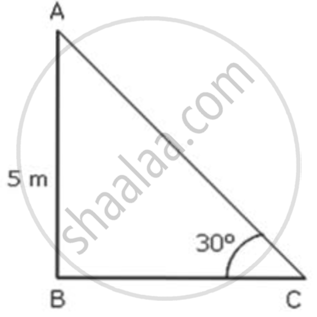Share

# The Top of a Ladder Reaches a Pcint on the Wall 5 M Above the Ground. If the Foot of the Ladder Makes an Angle of 30° with the Ground, Find the Length of the Ladder. - ICSE Class 10 - Mathematics

ConceptHeights and Distances - Solving 2-D Problems Involving Angles of Elevation and Depression Using Trigonometric Tables

#### Question

The top of a ladder reaches a pcint on the wall 5 m above the ground. If the foot of the ladder makes an angle of 30° with the ground, find the length of the ladder.

#### SolutionHere, AC is the ladder and AB is the point which is 5 m above the ground.
In ΔABC,

sin30° = "AB"/"AC"

⇒ 1/2 = 5/(AC)

⇒ AC = 10

Thus , the length of the ladder is 10 m.

Is there an error in this question or solution?
Solution The Top of a Ladder Reaches a Pcint on the Wall 5 M Above the Ground. If the Foot of the Ladder Makes an Angle of 30° with the Ground, Find the Length of the Ladder. Concept: Heights and Distances - Solving 2-D Problems Involving Angles of Elevation and Depression Using Trigonometric Tables.
S